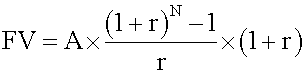Tags
Question
Future value of an annuity due

(without calculating the 2 parts)Tags
Question
Future value of an annuity due

(without calculating the 2 parts)
?

Tags
Question
Future value of an annuity due

(without calculating the 2 parts)If you want to change selection, open document below and click on "Move attachment"

Subject 4. The Future Value and Present Value of a Series of Equal Cash Flows (Ordinary Annuities, Annuity Dues, and Perpetuities)
sent value of a regular annuity Annuity due has a first cash flow that is paid immediately (indexed at t = 0). In other words, the payments occur at the beginning of each period. <span>Future value of an annuity due This consists of two parts: the future value of one annuity payment now, and the future value of a regular annuity of (N -1) period. Calculate the two parts and add them together. Alternatively, you can use this formula: Note that, all other factors being equal, the future value of an annuity due is equal to the future value of an ordinary annuity multiplied by

#### Summary

status measured difficulty not learned 37% [default] 0

No repetitions